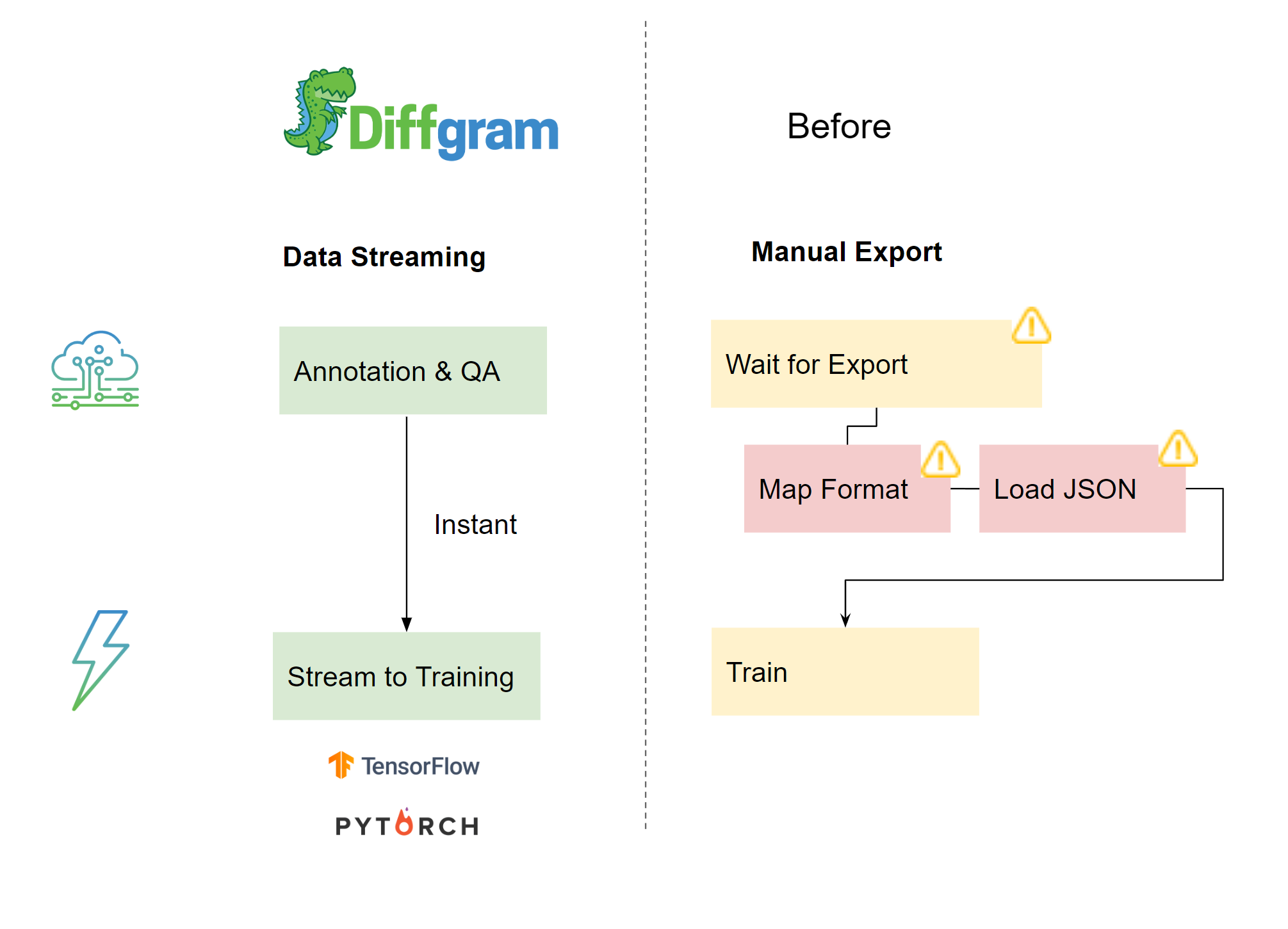# Streaming Export Introduction

Classically annotations were always exported into a static file. For example a JSON file.
Diffgram supports this: Export Walkthrough.

There is another new way. A new tool in your toolkit.

That's Streaming Data. Instead of generating an export as a one off thing, and then manually mapping that file, you stream the data directly into memory.If you are just getting started we encourage you to consider starting with Streaming, becuase then you can more easily expand into large production scale use cases. Exporting the file still works fine too, so alternatively just awareness of the availability of streaming can be good.

# Streaming Benefits

1. Load only what you need, which may be a small percent of a JSON file.
At scale it may be impractical to load all the data into a JSON file and so this may be a major benefit.
2. Works better for large teams. Avoids waiting for static files. You can program and do work with the expected dataset before Annotation starts, or even while Annotation is happening.
3. More memory efficient - becuase it's streaming, you need not ever load the entire dataset into memory. This is especially applicable for distributed training, and when marshalling a JSON file would be impractical on a local machine.
4. Saves having "double mapping" e.g. mapping to another format, which will itself then be mapped to tensors. In some cases too parsing a JSON file can take even more effort then just updating a few tensors.
5. More flexibility, no need for a pre-defined "format".

# Streaming Drawbacks

1. Defined in code. If the dataset changes may effect reproducibility unless additional steps are taken.
2. Requires understanding mapping the tensors.
3. Requires a network connection.
4. Some legacy training systems / AutoML providers may not support loading directly from memory and may require static files.

# Comparison Table

File

Streaming

Use cases

Small Datasets
Backup

Large Datasets

Generation

All at once

Per item (e.g per image)

Mapping

Load and map JSON file

Map tensors

Query

Load all data first,
then construct query after the fact

slice()

Integration

Manual
SDK/API

SDK/API

# Example

``````!pip install diffgram

from diffgram import Project

# Public project example
coco_project = Project(project_string_id='coco-dataset')
default_dataset = coco_project.directory.get(name = 'Default')
# Let's see how many images we got
print('Number of items in dataset: {}'.format(len(default_dataset)))

# Let's stream just the 8th element of the dataset
print('8th element: {}'.format(default_dataset))

# EXAMPLE tensor mapping
# See AdjustForRCNN() example below
)
"""
Example of a training data sample: a Tupple where first elm is the image and second elm is a dict with the label data.

(<PIL.Image.Image image mode=RGB size=650x417 at 0x7FE20F358908>,
{'area': tensor([141120.]),
'boxes': tensor([[ 81.,  88., 522., 408.]]),
'image_id': tensor(),
'iscrowd': tensor(),
'labels': tensor()}
)
"""
``````

Notebook example

# Example Process

1. Query the data desired with slice()
2. Map tensors as required with to_pytorch() or to_tensorflow()
3. Run it!

# Tensors Mapping Example

See the notebook for complete walkthrough Notebook example.

``````def get_transform():
transforms = []
transforms.append(AdjustForRCNN()) # Our previously created transform
# converts the image, a PIL image, into a PyTorch Tensor
transforms.append(T.ToTensor())
return ComposeTransforms(transforms)

"""
Adjust a sample from the Diffgram SDK generated pytorch
Dataset to be used for the RCNN model.

"""

def __call__(self, sample):
result = []
image = sample['image']
image_id = torch.tensor([sample['diffgram_file'].id])
project = sample['diffgram_file'].client
project.get_label_file_dict(use_session = False)
label_id_name_map = coco_project.name_to_file_id
label_id_list = [val for k, val in label_id_name_map.items()]
result.append(image)
boxes_array = []

for i in range(len(sample['x_min_list'])):
# Let's build the box array with the shape
# [x_min,y_min,x_max, y_max]
boxes_array.append([
sample['x_min_list'][i],
sample['y_min_list'][i],
sample['x_max_list'][i],
sample['y_max_list'][i],
])
boxes = torch.as_tensor(boxes_array, dtype = torch.float32)

num_objs = len(boxes_array)
labels_list = sample['label_id_list']
label_numbers_list = [label_id_list.index(x) for x in labels_list]
labels = torch.as_tensor(label_numbers_list, dtype = torch.int64)
if num_objs > 0:
area = (boxes[:, 3] - boxes[:, 1]) * (boxes[:, 2] - boxes[:, 0])
else:
area = torch.as_tensor([], dtype = torch.float32)
# suppose all instances are not crowd for this tutorial
iscrowd = torch.zeros((num_objs,), dtype = torch.int64)
target = {}
target["boxes"] = boxes
target["labels"] = labels
target["image_id"] = image_id
target["area"] = area
target["iscrowd"] = iscrowd
result.append(target)
image = result
target = result

return image, target
``````

# Context

There's two main reasons

1. It's a requirement for large use cases

2. Diffgram supports all media types, attributes etc. Even within the most well defined problem spaces, such as bounding box for images, there are multiple popular formats. When you consider say event level attributes on a Video the concept of a "format" becomes `undefined`. By refocusing the access around queries, the SDK, and so forth it help provide a best in class experience regardless of the complexity of the case.